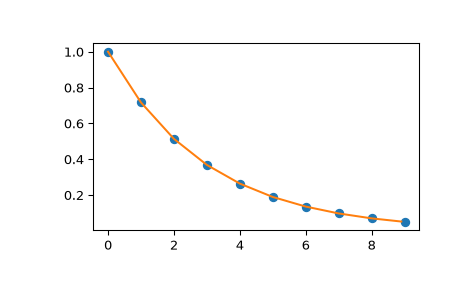# scipy.interpolate.interp1d¶

class scipy.interpolate.interp1d(x, y, kind='linear', axis=- 1, copy=True, bounds_error=None, fill_value=nan, assume_sorted=False)[source]

Interpolate a 1-D function.

x and y are arrays of values used to approximate some function f: `y = f(x)`. This class returns a function whose call method uses interpolation to find the value of new points.

Parameters
x(N,) array_like

A 1-D array of real values.

y(…,N,…) array_like

A N-D array of real values. The length of y along the interpolation axis must be equal to the length of x.

kindstr or int, optional

Specifies the kind of interpolation as a string or as an integer specifying the order of the spline interpolator to use. The string has to be one of ‘linear’, ‘nearest’, ‘nearest-up’, ‘zero’, ‘slinear’, ‘quadratic’, ‘cubic’, ‘previous’, or ‘next’. ‘zero’, ‘slinear’, ‘quadratic’ and ‘cubic’ refer to a spline interpolation of zeroth, first, second or third order; ‘previous’ and ‘next’ simply return the previous or next value of the point; ‘nearest-up’ and ‘nearest’ differ when interpolating half-integers (e.g. 0.5, 1.5) in that ‘nearest-up’ rounds up and ‘nearest’ rounds down. Default is ‘linear’.

axisint, optional

Specifies the axis of y along which to interpolate. Interpolation defaults to the last axis of y.

copybool, optional

If True, the class makes internal copies of x and y. If False, references to x and y are used. The default is to copy.

bounds_errorbool, optional

If True, a ValueError is raised any time interpolation is attempted on a value outside of the range of x (where extrapolation is necessary). If False, out of bounds values are assigned `fill_value`. By default, an error is raised unless `fill_value="extrapolate"`.

fill_valuearray-like or (array-like, array_like) or “extrapolate”, optional
• if a ndarray (or float), this value will be used to fill in for requested points outside of the data range. If not provided, then the default is NaN. The array-like must broadcast properly to the dimensions of the non-interpolation axes.

• If a two-element tuple, then the first element is used as a fill value for `x_new < x` and the second element is used for `x_new > x[-1]`. Anything that is not a 2-element tuple (e.g., list or ndarray, regardless of shape) is taken to be a single array-like argument meant to be used for both bounds as `below, above = fill_value, fill_value`.

New in version 0.17.0.

• If “extrapolate”, then points outside the data range will be extrapolated.

New in version 0.17.0.

assume_sortedbool, optional

If False, values of x can be in any order and they are sorted first. If True, x has to be an array of monotonically increasing values.

`splrep`, `splev`

Spline interpolation/smoothing based on FITPACK.

`UnivariateSpline`

An object-oriented wrapper of the FITPACK routines.

`interp2d`

2-D interpolation

Notes

Calling `interp1d` with NaNs present in input values results in undefined behaviour.

Input values x and y must be convertible to float values like int or float.

If the values in x are not unique, the resulting behavior is undefined and specific to the choice of kind, i.e., changing kind will change the behavior for duplicates.

Examples

```>>> import matplotlib.pyplot as plt
>>> from scipy import interpolate
>>> x = np.arange(0, 10)
>>> y = np.exp(-x/3.0)
>>> f = interpolate.interp1d(x, y)
```
```>>> xnew = np.arange(0, 9, 0.1)
>>> ynew = f(xnew)   # use interpolation function returned by `interp1d`
>>> plt.plot(x, y, 'o', xnew, ynew, '-')
>>> plt.show()
```Attributes
`fill_value`

The fill value.

Methods

 Evaluate the interpolant